# Collection of stamps

Jano, Rado, and Fero have created a collection of stamps in a ratio of 5: 6: 9. Two of them had 429 stamps together. How many stamps did their shared collection have?

Result

n =  780

#### Solution:Leave us a comment of example and its solution (i.e. if it is still somewhat unclear...):

Showing 0 comments:Be the first to comment!#### To solve this example are needed these knowledge from mathematics:

Need help calculate sum, simplify or multiply fractions? Try our fraction calculator.

## Next similar examples:

1. Holidays - on poolChildren's tickets to the swimming pool stands x € for an adult is € 2 more expensive. There was m children in the swimming pool and adults three times less. How many euros make treasurer for pool entry?
2. Pizza 5You have 2/4 of a pizza and you want to share it equally between 2 people how much pizza does each person get?
3. DigitsShow that if x, y, z are 3 consecutive nonzero digits, zyx-xyz = 198, where zyx and xyz are three-digit numbers created from x, y, z.
4. Three numbers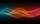The sum of the three numbers is 287. These numbers are in a ratio of 3: 7: 1/4 Define these numbers
5. Percentage 1052 shorts and missed 13. Calculate percentage
6. Ratio 11Simplify this ratio 10 : 1/4
7. PupilsThere are 32 pupils in the classroom, and girls are two-thirds more than boys. a) How many percents are more girls than boys? Round the result to a whole percentage. b) How many are boys in the class? c) Find the ratio of boys and girls in the class. Wr
8. Three machines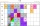The power of the three machines is 2: 3: 5. Two most powerful machines produce 400 parts per hour. How many components make all three machines in 3 hours?
9. College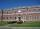At the college are at 57 rooms 194 students. Some rooms are triple and some quad. How many triple and quadruple rooms there is, if it is all fully occupied?
10. Life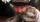Calculate how many years has man lived for 12248 days?
11. Lemonade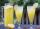How many 1/4 cup servings are in 2 and 1/3 cups of lemonade?
12. Unknown number 23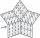Find 2/3 of unknown number, which is two thirds of the 99.
13. PipeSteel pipe has a length 2.5 meters. About how many decimetres is 1/3 less than 4/8 of this steel pipe?
14. Product of two fractionsProduct of two fractions is 9 3/5 . If one of the fraction is 9 3/7. Find the other fraction.
15. Liters od milkThe cylinder-shaped container contains 80 liters of milk. Milk level is 45 cm. How much milk will in the container, if level raise to height 72 cm?
16. Crates 2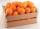One crate will hold 50 oranges. If Bob needs to ship 932 oranges, how many crates will he need?
17. Fractions 4How many 2/3s are in 6?